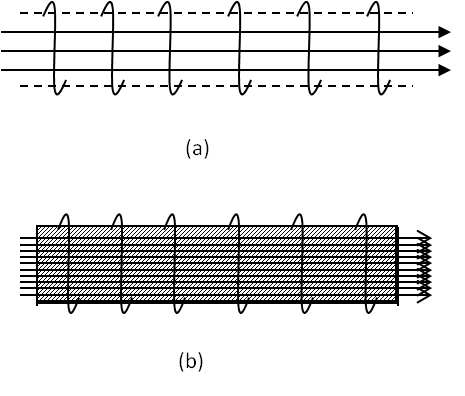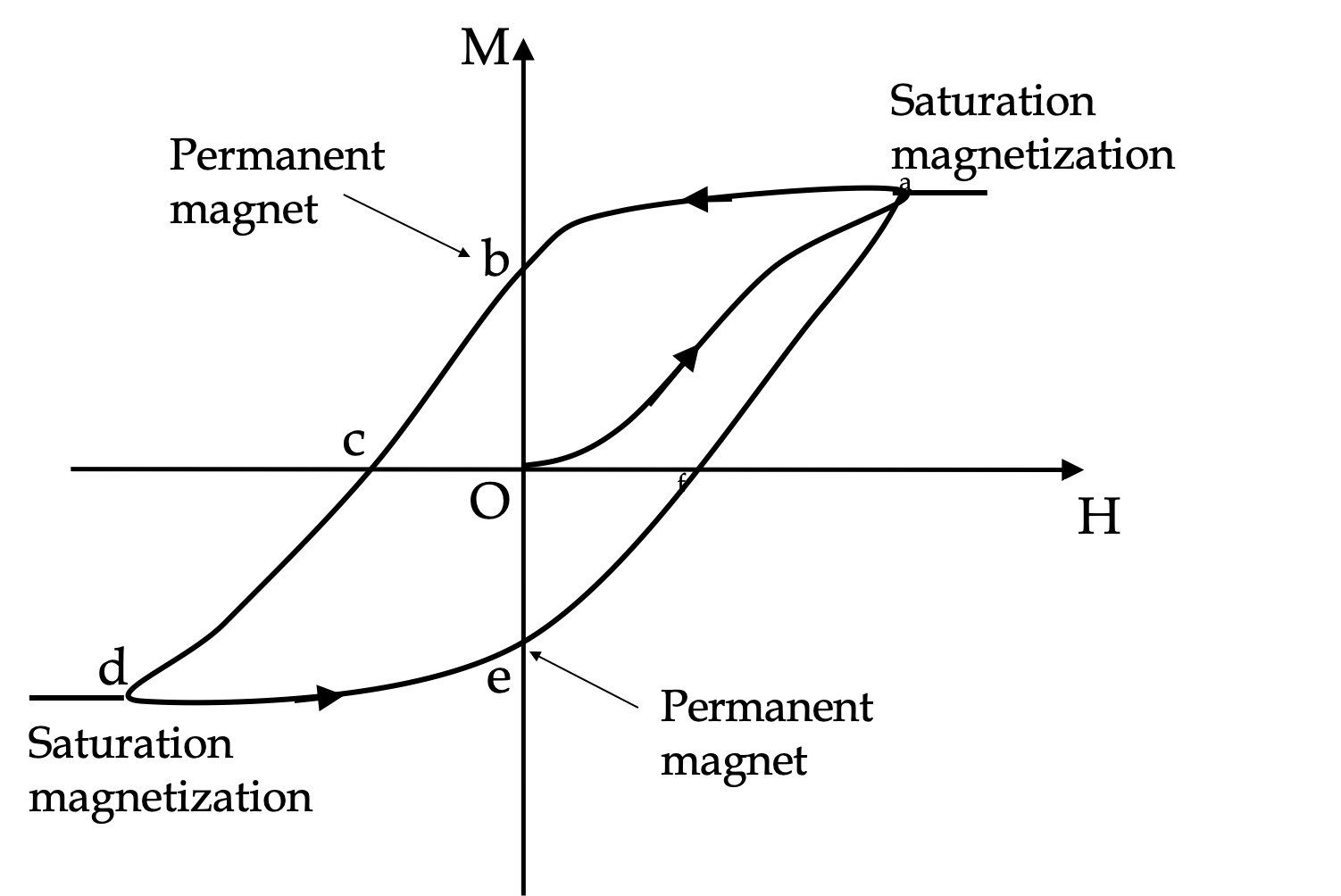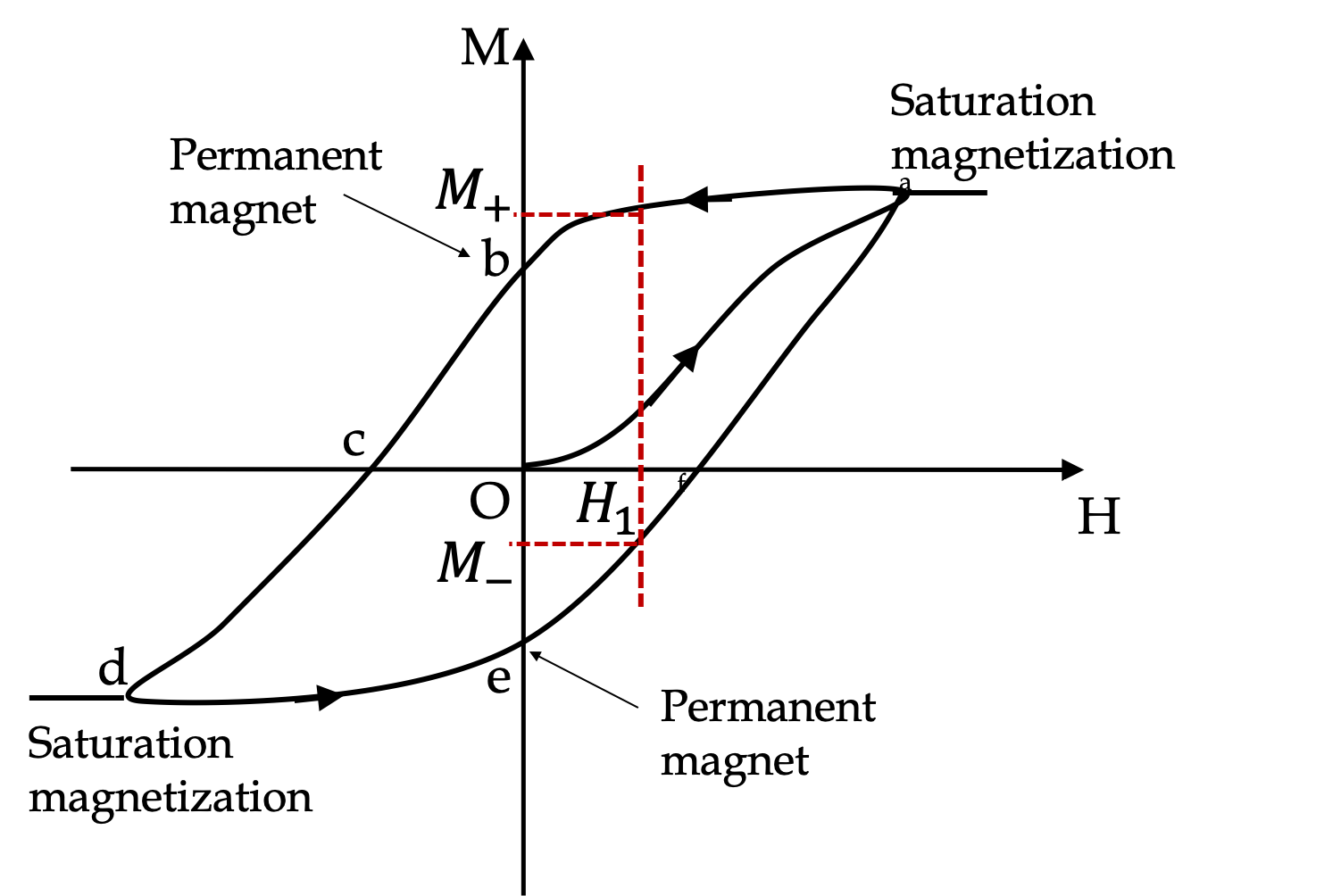## Section40.6Magnetization and Magnetic Field

Consider a solenoid obtained by winding a thin insulated copper wire over an iron core. When a current passes through the copper wire, magnetic field lines inside the solenoid pass through the iron core and align the atomic magnetic dipoles of iron, which produce additional magnetic field in the same direction. Thus, magnetic field inside the solenoid will be stronger with the iron core than without it.Figure 40.6.1. Solenoid without any core (a) and with iron core (b). Magnetic field inside the solenoid, $\vec B_\text{in}\text{,}$ is stronger with the iron core due to the sum of the fields by the current and that by the aligned magnetic dipoles of iron.

To understand the field when we have magnetic material, we separate external magnetic field from the magnetization-dependent field. Let $\vec H$ be the applied magnetic field, also called the magnetic auxliary field, and sometimes simply referred to as magnetic field when $\vec B$ is referred to as magnetic induction. Let $\vec M$ denote the magnetization, as before, then we write $\vec B/\mu_0$ as sum of $\vec H$ and $\vec M\text{.}$

\begin{equation} \dfrac{1}{\mu_0}\vec B = \vec H + \vec M.\label{eq-magnetic-auxiliary-field-definition}\tag{40.6.1} \end{equation}

Outside of magnetic material, where $M=0\text{,}$ we will have

\begin{equation} \dfrac{1}{\mu_0}\vec B_\text{out} = \vec H_\text{out}.\tag{40.6.2} \end{equation}

But, inside magnetic material, we will get

\begin{equation} \dfrac{1}{\mu_0}\vec B_\text{in} = \vec H_\text{in} + \vec M.\tag{40.6.3} \end{equation}

It can be shown that $\vec H_\text{in}$ obeys Ampere's law for the situation in the absence of any magnetic material. For Ampere's law symmetries, it is easy to find $\vec H_\text{in}\text{.}$ Thus, the problem of finding magnetic field $\vec B_\text{in}$ requires a way of knowing $\vec M\text{.}$ We will see below that this depends on whether the material is a linear magnetic material or non-linear.

### Subsection40.6.1Field in Paramagnets and Diamagnets

In paramagnets (such as Copper) and diamagnets (such as Bismuth), magnetization varies linearly with the applied field. This lets us define magnetic susceptibility $\chi_M$ of the material by

\begin{equation} \vec M = \chi_M \vec H,\label{eq-magnetic-susceptibility-definition}\tag{40.6.4} \end{equation}

where $H$ is the magnetic auxiliary field, which is proportional to the magnetic field $B$ inside the material.

\begin{equation} \vec H = \dfrac{1}{\mu} \vec B,\tag{40.6.5} \end{equation}

where $\mu$ is the magnetic permeability of the material. We can use the defining equation of $H\text{,}$ viz., Eq. (40.6.1), to note the relation between $\chi_M$ and $\mu$ of the material.

\begin{equation} \dfrac{\mu}{\mu_0} = 1 + \chi_M.\tag{40.6.6} \end{equation}

Using Eqs. (40.6.4) in Eq. (40.6.1) lets us find magnetic field $\vec B_\text{in}$ from a knowledge of extrernal field $\vec H_\text{in}$ and magnetic permeability.

\begin{equation} \vec B_\text{in} = \mu\, \vec H.\tag{40.6.7} \end{equation}

If external magnetic field is generated by simple current sources, it is easy to work out $H$ by using Ampere's law for $\vec H$ - this has the same form as that of $\vec B$ without the constant $\mu_0\text{.}$

### Subsection40.6.2(Calculus) Ampere's Law for Auxiliary Field

The auxiliary field obeys a law similar to the Ampere's law for $\vec B$ but with the current being only the real current, and not on the magnetic dipoles of the magnetic material.

\begin{equation} \oint \vec H\cdot d\vec l = I_\text{enc}^f\label{eq-amperes-law-auxiliary-field}\tag{40.6.8} \end{equation}

where $I^f$ denotes the current in the wire. Along with (40.6.8), auxiliary field must also obey

\begin{equation} \vec\nabla\cdot \vec H = - \vec\nabla\cdot \vec M,\tag{40.6.9} \end{equation}

which can be expressed as surface integral over the surface of the magnet.

\begin{equation*} \oint_S \vec H\cdot d\vec A = -\oint_S \vec M\cdot d\vec A. \end{equation*}

An example of Eq (40.6.8) to infintely long tightly-wound solenoid around a magnetic material is that inside the solenoid the $H$ field has the following magnitude.

\begin{equation*} H_\text{inside solenoid} = n I, \end{equation*}

where $n$ is number of turns per unit distance and $I$ is the current in the wire.

### Subsection40.6.3Field in Ferromagnets

Magnetic field in ferromagnets is also given by

\begin{equation*} \vec B_\text{in} = \mu_0 (\vec H_\text{in} + \vec M), \end{equation*}

but $M$ varies nonlinearly with $H$ in a complicated manner and you don't have a simple relation like

\begin{equation*} M \ne \chi_M H. \end{equation*}

Instead, the relation between $M$ and $H$ is much more complicated indicated in the hysteresis Figure 40.6.3. For the same field $H\text{,}$ you may get different values of magnetization. Not only that, $M$ value will also depend upon the magnetization history of the sample, i.e., immediately prior to a new $H$ what was the $H$ you had applied. So, how are we to get $B$ in a ferromagnetic material?Figure 40.6.3. Hysterisis of magnetization $M$ results when applied magnetic field $H$ is varied in a ferromagnetic material. Suppose you start with an unmagnetization material at $H=0\text{.}$ As you increase $H\text{,}$ say pointed towards $z$ axis, magnetization increases nonlinearly and reaches saturation point a. Now, you begin to decrease $H\text{,}$ you will return to $H=0$ at b, but magnetization does not return to zero. We say that we have magnetized the material. As you flip the direction of $H\text{,}$ i.e., now $H_z \lt 0\text{,}$ and increase its strength, you will need $H_z=H_c$ to make magnetization zero. If you continue to keep increasing th strength of $H\text{,}$ you would flip all dipoles at d amd get full magnetization in the $-z$ direction. Decreasing the strength of $H_z \lt 0$ field to zero again leaves the sample permanently magnetized, i.e., no external field but nonzero magnetization. The loop abcdefa is called a hysteresis loop. Note tha just knowing $H$ does not give you a unique value of $M\text{.}$ Hysterisis curve is different for different materials.

To find $B_\text{in}$ in a ferromagnet, we use the Hysterisis loop of the material to read possible value of $M$ and use the history of the smaple to pick the $M$ thats relevant for the situation at hand. For instance, as illustrated in Figure 40.6.4, if applied field is $H=H_1\text{,}$ any value of $M$ between $M_-$ and $M_+$ is posssible, depending upon history. You might say this is very frustrating when dealing with ferromagnets.Figure 40.6.4. History dependence of magnetization in a ferromagnet. For applied field $H=H_1\text{,}$ any value of $M$ between $M_-$ and $M_+$ is posssible, depending upon history.

A long copper rod of radius $R$ is carrying a current $I$ uniformly distributed over the cross-section.

(a) Find the magnitude and direction of the applied field $\vec H\text{,}$ magnetization $\vec M\text{,}$ and magnetic field $\vec B$ inside and outside the wire. Use $\chi_{M,\text{Cu}}$ for magnetic susceptibility of Copper.

(b) Find numerical values for $R=1\text{ cm}$ and $I=100\text{ A}$ at a point $0.5\text{ cm}$ from the center of the wire.

Data: $\chi_{M,\text{Cu}} = −0.97\times 10^{-5}\text{.}$

Hint

Find $\vec H$ first.

(a) Magnitudes at a point a distance $r\lt R$ from center : $H_{\textrm{in}} = J\: r/2\text{,}$ $M = \chi_m\: J\: r/2\text{,}$ $B_{\textrm{in}} = \mu_0\: (1+\chi_m)\: J\: r/2\text{.}$ Here $J= I/\pi R^2\text{.}$

(b) $5.0\times 10^{4}\text{ A/m}\text{,}$ $-4.85\times 10^{4}\text{ A/m}\text{,}$ $1.88\times 10^{-3}\text{ T}\text{.}$

Solution 1 (a)

(a) Let $s$ be the cylindrical radial distance from the center of the wire. Then, applying Ampere's law to the applied field $\vec H$ for the cylindrical symmetery, we immediately know that the magnitude $H$ is

\begin{equation*} H = \begin{cases} \dfrac{I}{R^2}\, s, \amp s \lt R\\ \dfrac{I}{2\pi s}, \amp s \gt R \end{cases} \end{equation*}

If you don't know how I got that, you will need to review Ampere's law in an earlier chapter. The direction of $\vec H$ will be tangent to circles around the wire, just as it was for $\vec B$ when we didn't worry about magnetization of copper itself.

Using susceptibility of Copper for points inside copper and permeability of vacuum at outside points, we get magnitude of $\vec M\text{.}$

\begin{equation*} M = \begin{cases} \dfrac{\chi_{M,\text{Cu}}\, I}{R^2}\, s, \amp s \lt R\\ 0, \amp s \gt R \end{cases} \end{equation*}

Since Cu is diamagnetic, its susceptibility $\chi_{\text{Cu},M} \lt 0\text{.}$ That means, the direction of $\vec M$ is opposite of the direction of $\vec H\text{.}$

Since $\vec B = \mu_0(\vec H + \vec M)$ we get $\vec B$ from the results given above. Alternaterly, we can use permeability of Copper for points inside copper and permeability of vacuum at outside points, we get magnitude of $\vec B\text{.}$

\begin{equation*} B = \begin{cases} \dfrac{\mu_\text{Cu}\, I}{R^2}\, s, \amp s \lt R\\ \dfrac{\mu_0\,I}{2\pi s}, \amp s \gt R \end{cases} \end{equation*}

with

\begin{equation*} \mu_\text{Cu} = 1 + \chi_{M,\text{Cu}}. \end{equation*}

The direction of $\vec B$ is same as the direction of $\vec H\text{.}$

Solution 2 (b)

(b) The magnitudes of the fields at the indicated point inside are

\begin{align*} H_\text{in} \amp = \dfrac{I}{R^2}\, s\\ \amp = \dfrac{100\text{ A}}{0.01^2\text{ m}^2}\, 0.05\text{ m} = 5.0\times 10^{4}\text{ A/m}. \\ M \amp = \chi_{M,\text{Cu}} H_\text{in} \\ \amp = −0.97\times 10^{-5} \times 5.0\times 10^{4}\text{ A/m} = -4.85\times 10^{4}\text{ A/m}\\ B \amp = \mu_0 (H + M)\\ \amp = 4\pi\times 10^{-7}\text{ T.m/A}\times (5.0-4.85)\times 10^{4} \text{ A/m}= 1.88\times 10^{-3}\text{ T}. \end{align*}

A solenoid is constructed by wounding wires over a long platinum rod. For a winding of $3000 \text{ turns per meter}$ and a current of $15\text{ A}$ through the wires, find the magnetization of platinum.

Data: $\chi_{M,\text{Pt}} = + 26.74\times 10^{-5}\text{.}$

Hint

Find $\vec H$ first.

Magnitude: $12.0\text{ A/m}\text{,}$ Direction: same as the magnetic field.

Solution

To ensure the symmetry we will assume that we have an infinitely long solenoid. With this assumption when we apply Ampere's law for $\vec H\text{,}$ we find the following for the magnitude $H$ at cylindrical radial distance $s$ from the axis, for solenoid of $n$ turns per unit length and current $I\text{.}$

\begin{equation*} H = \begin{cases} nI, \amp s \text{ inside}\\ 0, \amp s \text{ outside} \end{cases} \end{equation*}

The direction is given by the right-hand rule. Since platinum is paramagnetic, we can get $\vec M$ from $\vec H_\text{in}$ and $\chi_M\text{.}$

\begin{equation*} \vec M = \chi_M\, \vec H_\text{in}. \end{equation*}

Numerically, we will get

\begin{align*} M \amp = \chi_M\, nI \\ \amp = 26.74\times 10^{-5}\times 3000\text{ m}^{-1} \times 15\text{ A} =12.0\text{ A/m}. \end{align*}

Since $\chi_M$ of Pt is positive, $\vec M$ is in the same direction as $\vec H\text{.}$

Thin insulated wires run parallel in a water tank. There are $2500\text{ wires per meter}\text{,}$ each carrying the same current of $8\text{ A}\text{.}$ Find the magnetization of water.

Data: $\chi_{M,\text{H}_2\text{O}} = −9.035\times 10^{−6}.$

Hint

Treat current in wires as forming a surface current over water.

Magnitude: $9.035 \times 10^{-2} \text{ A/m}\text{,}$ Direction: Opposite to the magnetic field.

Solution

Parallel wires above water can be treated as planar current with surface current density $K\text{,}$

\begin{align*} \amp K = 2500\text{ m}^{-1} \times 8\text{ A} = 20,000\text{ A/m}.\\ \amp H = \dfrac{K}{2} = 10,000 \textrm{ A/m}. \end{align*}

Since water is a diamagnetic, we can get magnetization $\vec M$ from $\vec H$ and $\chi_M\text{.}$ Therefore magnetization of water under these conditions is

\begin{equation*} M = \chi_M\: H = −9.035\times 10^{−6}\times 10^4\textrm{ A/m} = −9.035 \times 10^{-2} \text{ A/m}. \end{equation*}

The sign is negative because magnetization is in opposite direction of $\vec H\text{.}$

A long tungsten cylindrical wire of thickness $2\text{ mm}$ is surrounded by oxygen gas at $1\text{ atm}$ and $20^{\circ}\text{C}$ in a glass tube. Oxygen gets magnetized when you pass current through the wire. But the magnetization depends upon distance from the wire. Find magnetization of oxygen at a distance $10\text{ cm}$ from the center of the wire when $3\text{ A}$ current passes in the wire.

Data: $\chi_{M,\text{O}_2} = 3.97\times 10^{-2}\text{.}$

Hint

Find $H$ first.

$1.9 \text{ A/m}\text{.}$

Solution

Let $s$ denote the cylindrical radial distance from the center of the wire. The applied field of the curren the wire, i.e., $\vec H$ has the following magnitude outside the wire.

\begin{equation*} H = \dfrac{I}{2\pi s} = \dfrac{0.48}{s}\: \textrm{A/m}, \end{equation*}

where $s$ is the numerical part of distance expressed in $\text{m}\text{.}$ The direction of $\vec H$ is tangent to circle about the wire. Since O$_2$ is paramagnetic, we can use $\chi_M$ to get $M$ at an arbitray point in O$_2\text{.}$

\begin{equation*} M = \chi_{M} H = 3.97\times 10^{-2} \times \dfrac{0.48}{s}\: \textrm{A/m} = \frac{1.9\times 10^{-2}}{s} \ \textrm{A/m}. \end{equation*}

Therefore, at $s=10\text{ cm}$ we will get

\begin{equation*} M = \frac{1.9\times 10^{-2}}{0.01 } \ \textrm{A/m} = 1.9 \text{ A/m}. \end{equation*}

the direction of $\vec M$ is same as that of $\vec H\text{.}$